x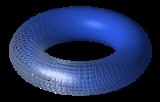TorusOverview

In geometry
Geometry
Geometry arose as the field of knowledge dealing with spatial relationships. Geometry was one of the two fields of pre-modern mathematics, the other being the study of numbers ....

, a torus (pl. tori) is a surface of revolution
Surface of revolution
A surface of revolution is a surface in Euclidean space created by rotating a curve around a straight line in its plane ....

generated by revolving a circle
Circle
A circle is a simple shape of Euclidean geometry consisting of those points in a plane that are a given distance from a given point, the centre. The distance between any of the points and the centre is called the radius....

in three dimensional space about an axis coplanar with the circle. In most contexts it is assumed that the axis does not touch the circle - in this case the surface has a ring shape and is called a ring torus or simply torus if the ring shape is implicit.

Other types of torus include the horn torus, which is generated when the axis is tangent to the circle, and the spindle torus, which is generated when the axis is a chord of the circle.Unanswered QuestionsWith tori showing up so often in astrophysics and cosmology, has an exact exterior metric for a rapidly rotating (but non-black hole) torus (with it s...when we do surgical removal for the torus mandibularis?Encyclopedia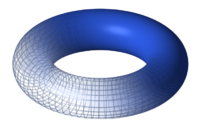In geometry
Geometry
Geometry arose as the field of knowledge dealing with spatial relationships. Geometry was one of the two fields of pre-modern mathematics, the other being the study of numbers ....

, a torus (pl. tori) is a surface of revolution
Surface of revolution
A surface of revolution is a surface in Euclidean space created by rotating a curve around a straight line in its plane ....

generated by revolving a circle
Circle
A circle is a simple shape of Euclidean geometry consisting of those points in a plane that are a given distance from a given point, the centre. The distance between any of the points and the centre is called the radius....

in three dimensional space about an axis coplanar with the circle. In most contexts it is assumed that the axis does not touch the circle - in this case the surface has a ring shape and is called a ring torus or simply torus if the ring shape is implicit.

Other types of torus include the horn torus, which is generated when the axis is tangent to the circle, and the spindle torus, which is generated when the axis is a chord of the circle. A degenerate
Degeneracy (mathematics)
In mathematics, a degenerate case is a limiting case in which a class of object changes its nature so as to belong to another, usually simpler, class....

case is when the axis is a diameter of the circle, which simply generates the surface of a sphere
Sphere
A sphere is a perfectly round geometrical object in three-dimensional space, such as the shape of a round ball. Like a circle in two dimensions, a perfect sphere is completely symmetrical around its center, with all points on the surface lying the same distance r from the center point...

. The ring torus bounds a solid known as a toroid. The adjective toroidal can be applied to tori, toroids or, more generally, any ring shape as in toroidal inductors and transformers
Toroidal inductors and transformers
Toroidal inductors and transformers are electronic components, typically consisting of a circular ring-shaped magnetic core of iron powder, ferrite, or other material around which wire is coiled to make an inductor. Toroidal coils are used in a broad range of applications, such as high-frequency...

. Real world examples of (approximately) toroidal objects include doughnut
Doughnut
A doughnut or donut is a fried dough food and is popular in many countries and prepared in various forms as a sweet snack that can be homemade or purchased in bakeries, supermarkets, food stalls, and franchised specialty outlets...

s, vadais, inner tubes, many lifebuoy
Lifebuoy
A lifebuoy, ring buoy, lifering, lifesaver, life preserver or lifebelt, also known as a "kisby ring" or "perry buoy", is a life saving buoy designed to be thrown to a person in the water, to provide buoyancy, to prevent drowning...

s, O-ring
O-ring
An O-ring, also known as a packing, or a toric joint, is a mechanical gasket in the shape of a torus; it is a loop of elastomer with a disc-shaped cross-section, designed to be seated in a groove and compressed during assembly between two or more parts, creating a seal at the interface.The O-ring...

s and vortex ring
Vortex ring
A vortex ring, also called a toroidal vortex, is a region of rotating fluid moving through the same or different fluid where the flow pattern takes on a toroidal shape. The movement of the fluid is about the poloidal or circular axis of the doughnut, in a twisting vortex motion...

s.

In topology
Topology
Topology is a major area of mathematics concerned with properties that are preserved under continuous deformations of objects, such as deformations that involve stretching, but no tearing or gluing...

, a ring torus is homeomorphic
Homeomorphism
In the mathematical field of topology, a homeomorphism or topological isomorphism or bicontinuous function is a continuous function between topological spaces that has a continuous inverse function. Homeomorphisms are the isomorphisms in the category of topological spaces—that is, they are...

to the Cartesian product
Product topology
In topology and related areas of mathematics, a product space is the cartesian product of a family of topological spaces equipped with a natural topology called the product topology...

of two circle
Circle
A circle is a simple shape of Euclidean geometry consisting of those points in a plane that are a given distance from a given point, the centre. The distance between any of the points and the centre is called the radius....

s: S1 × S1, and the latter is taken to be the definition in that context. It is a compact 2-manifold of genus 1. The ring torus is one way to embed this space into Euclidean space, but another way to do this is the Cartesian product of the embedding of S1 in the plane. This produces a geometric object called the Clifford torus
Clifford torus
In geometric topology, the Clifford torus is a special kind of torus sitting inside R4. Alternatively, it can be seen as a torus sitting inside C2 since C2 is topologically the same space as R4...

, surface in 4-space.

The word torus comes from the Latin
Latin
Latin is an Italic language originally spoken in Latium and Ancient Rome. It, along with most European languages, is a descendant of the ancient Proto-Indo-European language. Although it is considered a dead language, a number of scholars and members of the Christian clergy speak it fluently, and...

word meaning cushion
Cushion
A cushion is a soft bag of some ornamental material, stuffed with wool, hair, feathers, polyester staple fiber, non-woven material, or even paper torn into fragments. It may be used for sitting or kneeling upon, or to soften the hardness or angularity of a chair or couch...

.

## Geometry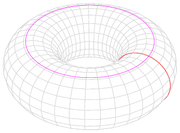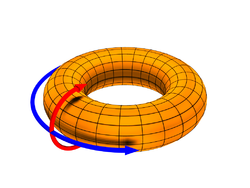A torus can be defined parametric
Parametric equation
In mathematics, parametric equation is a method of defining a relation using parameters. A simple kinematic example is when one uses a time parameter to determine the position, velocity, and other information about a body in motion....

ally by: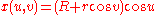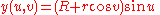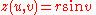where
u,v are in the interval [0, 2π),
R (or A) is the distance from the center of the tube to the center of the torus,
r (or a) is the radius of the tube.

R and r are also known as the "major radius" and "minor radius", respectively. The ratio of the two is known as the "aspect ratio
Aspect ratio
The aspect ratio of a shape is the ratio of its longer dimension to its shorter dimension. It may be applied to two characteristic dimensions of a three-dimensional shape, such as the ratio of the longest and shortest axis, or for symmetrical objects that are described by just two measurements,...

". A doughnut has an aspect ratio of 2 to 3.

An implicit
Implicit function
The implicit function theorem provides a link between implicit and explicit functions. It states that if the equation R = 0 satisfies some mild conditions on its partial derivatives, then one can in principle solve this equation for y, at least over some small interval...

equation in Cartesian coordinates for a torus radially symmetric about the z-axis is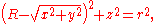or the solution of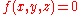, where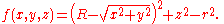Algebraically eliminating the square root gives a quartic equation,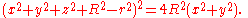The three different classes of standard tori correspond to the three possible relative sizes of r and R. When R > r, the surface will be the familiar ring torus. The case R = r corresponds to the horn torus, which in effect is a torus with no "hole". The case R < r describes the self-intersecting spindle torus. When R = 0, the torus degenerates to the sphere.

The surface area
Surface area
Surface area is the measure of how much exposed area a solid object has, expressed in square units. Mathematical description of the surface area is considerably more involved than the definition of arc length of a curve. For polyhedra the surface area is the sum of the areas of its faces...

and interior volume
Volume
Volume is the quantity of three-dimensional space enclosed by some closed boundary, for example, the space that a substance or shape occupies or contains....

of this torus are easily computed using Pappus's centroid theorem
Pappus's centroid theorem
In mathematics, Pappus' centroid theorem is either of two related theorems dealing with the surface areas and volumes of surfaces and solids of revolution....

giving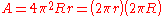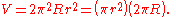These formulas are the same as for a cylinder of length 2πR and radius r, created by cutting the tube and unrolling it by straightening out the line running around the center of the tube. The losses in surface area and volume on the inner side of the tube exactly cancel out the gains on the outer side.

As a torus is the product of two circles, a modified version of the spherical coordinate system
Spherical coordinate system
In mathematics, a spherical coordinate system is a coordinate system for three-dimensional space where the position of a point is specified by three numbers: the radial distance of that point from a fixed origin, its inclination angle measured from a fixed zenith direction, and the azimuth angle of...

is sometimes used. In traditional spherical coordinates there are three measures, R, the distance from the center of the coordinate system, and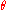and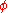, angles measured from the center point. As a torus has, effectively, two center points, the centerpoints of the angles are moved;measures the same angle as it does in the spherical system, but is known as the "toroidal" direction. The center point ofis moved to the center of r, and is known as the "poloidal" direction. These terms were first used in a discussion of the Earth's magnetic field, where "poloidal" was used to denote "the direction toward the poles". In modern use these terms are more commonly used to discuss magnetic confinement fusion
Magnetic confinement fusion
Magnetic confinement fusion is an approach to generating fusion power that uses magnetic fields to confine the hot fusion fuel in the form of a plasma. Magnetic confinement is one of two major branches of fusion energy research, the other being inertial confinement fusion. The magnetic approach is...

devices.

## Topology

Topologically
Topology
Topology is a major area of mathematics concerned with properties that are preserved under continuous deformations of objects, such as deformations that involve stretching, but no tearing or gluing...

, a torus is a closed surface
Surface
In mathematics, specifically in topology, a surface is a two-dimensional topological manifold. The most familiar examples are those that arise as the boundaries of solid objects in ordinary three-dimensional Euclidean space R3 — for example, the surface of a ball...

defined as the product
Product topology
In topology and related areas of mathematics, a product space is the cartesian product of a family of topological spaces equipped with a natural topology called the product topology...

of two circle
Circle
A circle is a simple shape of Euclidean geometry consisting of those points in a plane that are a given distance from a given point, the centre. The distance between any of the points and the centre is called the radius....

s: S1 × S1. This can be viewed as lying in C2 and is a subset of the 3-sphere S3 of radius √2. This topological torus is also often called the Clifford torus
Clifford torus
In geometric topology, the Clifford torus is a special kind of torus sitting inside R4. Alternatively, it can be seen as a torus sitting inside C2 since C2 is topologically the same space as R4...

. In fact, S3 is filled out
Foliation
In mathematics, a foliation is a geometric device used to study manifolds, consisting of an integrable subbundle of the tangent bundle. A foliation looks locally like a decomposition of the manifold as a union of parallel submanifolds of smaller dimension....

by a family of nested tori in this manner (with two degenerate circles), a fact which is important in the study of S3 as a fiber bundle
Fiber bundle
In mathematics, and particularly topology, a fiber bundle is intuitively a space which locally "looks" like a certain product space, but globally may have a different topological structure...

over S2 (the Hopf bundle
Hopf bundle
In the mathematical field of topology, the Hopf fibration describes a 3-sphere in terms of circles and an ordinary sphere. Discovered by Heinz Hopf in 1931, it is an influential early example of a fiber bundle...

).

The surface described above, given the relative topology from R3, is homeomorphic to a topological torus as long as it does not intersect its own axis. A particular homeomorphism is given by stereographically projecting
Stereographic projection
The stereographic projection, in geometry, is a particular mapping that projects a sphere onto a plane. The projection is defined on the entire sphere, except at one point — the projection point. Where it is defined, the mapping is smooth and bijective. It is conformal, meaning that it...

the topological torus into R3 from the north pole of S3.

The torus can also be described as a quotient
Quotient space
In topology and related areas of mathematics, a quotient space is, intuitively speaking, the result of identifying or "gluing together" certain points of a given space. The points to be identified are specified by an equivalence relation...

of the Cartesian plane under the identifications ~ (x+1,y) ~ (x,y+1).
Or, equivalently, as the quotient of the unit square
Unit square
In mathematics, a unit square is a square whose sides have length 1. Often, "the" unit square refers specifically to the square in the Cartesian plane with corners at , , , and .-In the real plane:...

by pasting the opposite edges together, described as a fundamental polygon
Fundamental polygon
In mathematics, each closed surface in the sense of geometric topology can be constructed from an even-sided oriented polygon, called a fundamental polygon, by pairwise identification of its edges....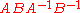.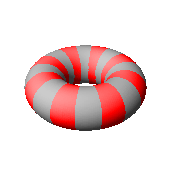The fundamental group
Fundamental group
In mathematics, more specifically algebraic topology, the fundamental group is a group associated to any given pointed topological space that provides a way of determining when two paths, starting and ending at a fixed base point, can be continuously deformed into each other...

of the torus is just the direct product
Direct product of groups
In the mathematical field of group theory, the direct product is an operation that takes two groups and and constructs a new group, usually denoted...

of the fundamental group of the circle with itself: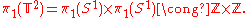Intuitively speaking, this means that a closed path
Path (topology)
In mathematics, a path in a topological space X is a continuous map f from the unit interval I = [0,1] to XThe initial point of the path is f and the terminal point is f. One often speaks of a "path from x to y" where x and y are the initial and terminal points of the path...

that circles the torus' "hole" (say, a circle that traces out a particular latitude) and then circles the torus' "body" (say, a circle that traces out a particular longitude) can be deformed to a path that circles the body and then the hole. So, strictly 'latitudinal' and strictly 'longitudinal' paths commute. This might be imagined as two shoelaces passing through each other, then unwinding, then rewinding.

If a torus is punctured and turned inside out then another torus results, with lines of latitude and longitude interchanged.

The first homology group of the torus is isomorphic to the fundamental group (this follows from Hurewicz theorem
Hurewicz theorem
In mathematics, the Hurewicz theorem is a basic result of algebraic topology, connecting homotopy theory with homology theory via a map known as the Hurewicz homomorphism...

since the fundamental group is abelian
Abelian group
In abstract algebra, an abelian group, also called a commutative group, is a group in which the result of applying the group operation to two group elements does not depend on their order . Abelian groups generalize the arithmetic of addition of integers...

).

## Two-sheeted cover

The 2-torus double-covers the 2-sphere, with four ramification points. Every conformal structure on the 2-torus can be represented as a two-sheeted cover of the 2-sphere. The points on the torus corresponding to the ramification points are the Weierstrass point
Weierstrass point
In mathematics, a Weierstrass point P on a nonsingular algebraic curve C defined over the complex numbers is a point such that there are extra functions on C, with their poles restricted to P only, than would be predicted by looking at the Riemann–Roch theorem...

s. In fact, the conformal type of the torus is determined by the cross-ratio
Cross-ratio
In geometry, the cross-ratio, also called double ratio and anharmonic ratio, is a special number associated with an ordered quadruple of collinear points, particularly points on a projective line...

of the four points.

## n-dimensional torus

The torus has a generalization to higher dimensions, the n-dimensional torus, often called the n-torus for short. (This is one of two different meanings of the term "n-torus".)
Recalling that the torus is the product space of two circles, the n-dimensional torus is the product of n circles.
That is: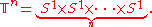The torus discussed above is the 2-dimensional torus. The 1-dimensional torus is just the circle. Just as for the 2-torus, the n-torus can be described as a quotient of Rn under integral shifts in any coordinate. That is, the n-torus is Rn modulo the action
Group action
In algebra and geometry, a group action is a way of describing symmetries of objects using groups. The essential elements of the object are described by a set, and the symmetries of the object are described by the symmetry group of this set, which consists of bijective transformations of the set...

of the integer lattice
Lattice (group)
In mathematics, especially in geometry and group theory, a lattice in Rn is a discrete subgroup of Rn which spans the real vector space Rn. Every lattice in Rn can be generated from a basis for the vector space by forming all linear combinations with integer coefficients...

Zn (with the action being taken as vector addition). Equivalently, the n-torus is obtained from the n-dimensional hypercube
Hypercube
In geometry, a hypercube is an n-dimensional analogue of a square and a cube . It is a closed, compact, convex figure whose 1-skeleton consists of groups of opposite parallel line segments aligned in each of the space's dimensions, perpendicular to each other and of the same length.An...

by gluing the opposite faces together.

An n-torus in this sense is an example of an n-dimensional compact
Compact space
In mathematics, specifically general topology and metric topology, a compact space is an abstract mathematical space whose topology has the compactness property, which has many important implications not valid in general spaces...

manifold
Manifold
In mathematics , a manifold is a topological space that on a small enough scale resembles the Euclidean space of a specific dimension, called the dimension of the manifold....

. It is also an example of a compact abelian
Abelian group
In abstract algebra, an abelian group, also called a commutative group, is a group in which the result of applying the group operation to two group elements does not depend on their order . Abelian groups generalize the arithmetic of addition of integers...

Lie group
Lie group
In mathematics, a Lie group is a group which is also a differentiable manifold, with the property that the group operations are compatible with the smooth structure...

. This follows from the fact that the unit circle
Unit circle
In mathematics, a unit circle is a circle with a radius of one. Frequently, especially in trigonometry, "the" unit circle is the circle of radius one centered at the origin in the Cartesian coordinate system in the Euclidean plane...

is a compact abelian Lie group (when identified with the unit complex number
Complex number
A complex number is a number consisting of a real part and an imaginary part. Complex numbers extend the idea of the one-dimensional number line to the two-dimensional complex plane by using the number line for the real part and adding a vertical axis to plot the imaginary part...

s with multiplication). Group multiplication on the torus is then defined by coordinate-wise multiplication.

Toroidal groups play an important part in the theory of compact Lie groups. This is due in part to the fact that in any compact Lie group G one can always find a maximal torus
Maximal torus
In the mathematical theory of compact Lie groups a special role is played by torus subgroups, in particular by the maximal torus subgroups.A torus in a Lie group G is a compact, connected, abelian Lie subgroup of G . A maximal torus is one which is maximal among such subgroups...

; that is, a closed subgroup
Subgroup
In group theory, given a group G under a binary operation *, a subset H of G is called a subgroup of G if H also forms a group under the operation *. More precisely, H is a subgroup of G if the restriction of * to H x H is a group operation on H...

which is a torus of the largest possible dimension. Such maximal tori T have a controlling role to play in theory of connected G.

Automorphism
Automorphism
In mathematics, an automorphism is an isomorphism from a mathematical object to itself. It is, in some sense, a symmetry of the object, and a way of mapping the object to itself while preserving all of its structure. The set of all automorphisms of an object forms a group, called the automorphism...

s of T are easily constructed from automorphisms of the lattice Zn, which are classified by integral matrices M of size n×n which are invertible with integral inverse; these are just the integral M of determinant +1 or −1. Making M act on Rn in the usual way, one has the typical toral automorphism on the quotient.

The fundamental group
Fundamental group
In mathematics, more specifically algebraic topology, the fundamental group is a group associated to any given pointed topological space that provides a way of determining when two paths, starting and ending at a fixed base point, can be continuously deformed into each other...

of an n-torus is a free abelian group
Free abelian group
In abstract algebra, a free abelian group is an abelian group that has a "basis" in the sense that every element of the group can be written in one and only one way as a finite linear combination of elements of the basis, with integer coefficients. Hence, free abelian groups over a basis B are...

of rank n. The k-th homology group of an n-torus is a free abelian group of rank n choose
Binomial coefficient
In mathematics, binomial coefficients are a family of positive integers that occur as coefficients in the binomial theorem. They are indexed by two nonnegative integers; the binomial coefficient indexed by n and k is usually written \tbinom nk , and it is the coefficient of the x k term in...

k. It follows that the Euler characteristic
Euler characteristic
In mathematics, and more specifically in algebraic topology and polyhedral combinatorics, the Euler characteristic is a topological invariant, a number that describes a topological space's shape or structure regardless of the way it is bent...

of the n-torus is 0 for all n. The cohomology ring
Cohomology ring
In mathematics, specifically algebraic topology, the cohomology ring of a topological space X is a ring formed from the cohomology groups of X together with the cup product serving as the ring multiplication. Here 'cohomology' is usually understood as singular cohomology, but the ring structure is...

H(Tn,Z) can be identified with the exterior algebra
Exterior algebra
In mathematics, the exterior product or wedge product of vectors is an algebraic construction used in Euclidean geometry to study areas, volumes, and their higher-dimensional analogs...

over the Z-module
Module (mathematics)
In abstract algebra, the concept of a module over a ring is a generalization of the notion of vector space, wherein the corresponding scalars are allowed to lie in an arbitrary ring...

Zn whose generators are the duals of the n nontrivial cycles.

### Configuration space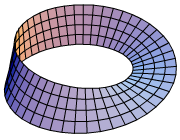As the n-torus is the n-fold product of the circle, the n-torus is the configuration space
Configuration space
- Configuration space in physics :In classical mechanics, the configuration space is the space of possible positions that a physical system may attain, possibly subject to external constraints...

of n ordered, not necessarily distinct points on the circle. Symbolically,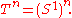The configuration space of unordered, not necessarily distinct points is accordingly the orbifold
Orbifold
In the mathematical disciplines of topology, geometry, and geometric group theory, an orbifold is a generalization of a manifold...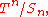which is the quotient of the torus by the symmetric group
Symmetric group
In mathematics, the symmetric group Sn on a finite set of n symbols is the group whose elements are all the permutations of the n symbols, and whose group operation is the composition of such permutations, which are treated as bijective functions from the set of symbols to itself...

on n letters (by permuting the coordinates).

For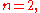the quotient is the Möbius strip
Möbius strip
The Möbius strip or Möbius band is a surface with only one side and only one boundary component. The Möbius strip has the mathematical property of being non-orientable. It can be realized as a ruled surface...

, the edge corresponding to the orbifold points where the two coordinates coincide. For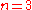this quotient may be described as a solid torus with cross-section an equilateral triangle, with a twist; equivalently, as a triangular prism
Triangular prism
In geometry, a triangular prism is a three-sided prism; it is a polyhedron made of a triangular base, a translated copy, and 3 faces joining corresponding sides....

whose top and bottom faces are connected with a ⅓ twist (120°): the 3-dimensional interior corresponds to the points on the 3-torus where all 3 coordinates are distinct, the 2-dimensional face corresponds to points with 2 coordinates equal and the 3rd different, while the 1-dimensional edge corresponds to points with all 3 coordinates identical.

These orbifolds have found significant applications to music theory in the work of Dmitri Tymoczko and collaborators (Felipe Posada and Michael Kolinas, et al.), being used to model musical triad
Triad (music)
In music and music theory, a triad is a three-note chord that can be stacked in thirds. Its members, when actually stacked in thirds, from lowest pitched tone to highest, are called:* the Root...

s.

## Flat torus

The flat torus is a specific embedding of the familiar 2-torus into Euclidean 4-space or higher dimensions. Its surface has zero Gaussian curvature
Gaussian curvature
In differential geometry, the Gaussian curvature or Gauss curvature of a point on a surface is the product of the principal curvatures, κ1 and κ2, of the given point. It is an intrinsic measure of curvature, i.e., its value depends only on how distances are measured on the surface, not on the way...

everywhere. Its surface is "flat" in the same sense that the surface of a cylinder is "flat". In 3 dimensions one can bend a flat sheet of paper into a cylinder without stretching the paper, but you cannot then bend this cylinder into a torus without stretching the paper. In 4 dimensions one can (mathematically).

A simple 4-d Euclidean embedding is as follows: <x,y,z,w> = <R cos u, R sin u, P cos v, P sin v> where R and P
are constants determining the aspect ratio. It is diffeomorphic to a regular torus but not isometric
Isometry
In mathematics, an isometry is a distance-preserving map between metric spaces. Geometric figures which can be related by an isometry are called congruent.Isometries are often used in constructions where one space is embedded in another space...

. It can not be isometrically embedded into Euclidean 3-space. Mapping
Map (mathematics)
In most of mathematics and in some related technical fields, the term mapping, usually shortened to map, is either a synonym for function, or denotes a particular kind of function which is important in that branch, or denotes something conceptually similar to a function.In graph theory, a map is a...

it into 3-space requires you to "bend" it, in which
case it looks like a regular torus, for example, the following map <x,y,z> = <(R + P sin v)cos u, (R + P sin v)sin u, P cos v>.

A flat torus partitions the 3-sphere
3-sphere
In mathematics, a 3-sphere is a higher-dimensional analogue of a sphere. It consists of the set of points equidistant from a fixed central point in 4-dimensional Euclidean space...

into two congruent solid tori subsets with the aforsaid flat torus surface as their common boundary
Boundary (topology)
In topology and mathematics in general, the boundary of a subset S of a topological space X is the set of points which can be approached both from S and from the outside of S. More precisely, it is the set of points in the closure of S, not belonging to the interior of S. An element of the boundary...

.
 A stereographic projection of a Clifford torusClifford torusIn geometric topology, the Clifford torus is a special kind of torus sitting inside R4. Alternatively, it can be seen as a torus sitting inside C2 since C2 is topologically the same space as R4... performing a simple rotation through the xz-plane.

## n-fold torus

In the theory of surface
Surface
In mathematics, specifically in topology, a surface is a two-dimensional topological manifold. The most familiar examples are those that arise as the boundaries of solid objects in ordinary three-dimensional Euclidean space R3 — for example, the surface of a ball...

s the term n-torus has a different meaning. Instead of the product of n circles, they use the phrase to mean the connected sum
Connected sum
In mathematics, specifically in topology, the operation of connected sum is a geometric modification on manifolds. Its effect is to join two given manifolds together near a chosen point on each...

of n 2-dimensional tori. To form a connected sum of two surfaces, remove from each the interior of a disk and "glue" the surfaces together along the disks' boundary circles. To form the connected sum of more than two surfaces, sum two of them at a time until they are all connected together. In this sense, an n-torus resembles the surface of n doughnuts stuck together side by side, or a 2-dimensional sphere
Sphere
A sphere is a perfectly round geometrical object in three-dimensional space, such as the shape of a round ball. Like a circle in two dimensions, a perfect sphere is completely symmetrical around its center, with all points on the surface lying the same distance r from the center point...

with n handles attached.

An ordinary torus is a 1-torus, a 2-torus is called a double torus
Double torus
In mathematics, a genus-2 surface is a surface formed by the connected sum of two tori. That is to say, from each of two tori the interior of a disk is removed, and the boundaries of the two disks are identified , forming a double torus.This is the simplest case of the connected sum of n tori...

, a 3-torus a triple torus
Triple torus
Triple torus or three-torus can refer to one of the two following concepts, both related to a torus.-Three-dimensional torus:The three-dimensional torus, or triple torus, is defined as the Cartesian product of three circles,...

, and so on. The n-torus is said to be an "orientable surface
Orientability
In mathematics, orientability is a property of surfaces in Euclidean space measuring whether or not it is possible to make a consistent choice of surface normal vector at every point. A choice of surface normal allows one to use the right-hand rule to define a "clockwise" direction of loops in the...

" of "genus
Genus (mathematics)
In mathematics, genus has a few different, but closely related, meanings:-Orientable surface:The genus of a connected, orientable surface is an integer representing the maximum number of cuttings along non-intersecting closed simple curves without rendering the resultant manifold disconnected. It...

" n, the genus being the number of handles. The 0-torus is the 2-dimensional sphere
Sphere
A sphere is a perfectly round geometrical object in three-dimensional space, such as the shape of a round ball. Like a circle in two dimensions, a perfect sphere is completely symmetrical around its center, with all points on the surface lying the same distance r from the center point...

.

The classification theorem
Classification theorem
In mathematics, a classification theorem answers the classification problem "What are the objects of a given type, up to some equivalence?". It gives a non-redundant enumeration: each object is equivalent to exactly one class....

for surfaces states that every compact
Compact space
In mathematics, specifically general topology and metric topology, a compact space is an abstract mathematical space whose topology has the compactness property, which has many important implications not valid in general spaces...

connected
Connected space
In topology and related branches of mathematics, a connected space is a topological space that cannot be represented as the union of two or more disjoint nonempty open subsets. Connectedness is one of the principal topological properties that is used to distinguish topological spaces...

surface is either a sphere, an n-torus with n > 0, or the connected sum of n projective plane
Projective plane
In mathematics, a projective plane is a geometric structure that extends the concept of a plane. In the ordinary Euclidean plane, two lines typically intersect in a single point, but there are some pairs of lines that do not intersect...

s (that is, projective planes over the real numbers) with n > 0.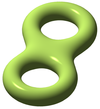double torusDouble torusIn mathematics, a genus-2 surface is a surface formed by the connected sum of two tori. That is to say, from each of two tori the interior of a disk is removed, and the boundaries of the two disks are identified , forming a double torus.This is the simplest case of the connected sum of n tori...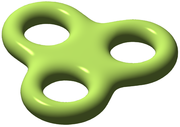triple torusTriple torusTriple torus or three-torus can refer to one of the two following concepts, both related to a torus.-Three-dimensional torus:The three-dimensional torus, or triple torus, is defined as the Cartesian product of three circles,...

## Toroidal polyhedra

Polyhedra with the topological type of a torus are called toroidal polyhedra, and satisfy a modified version of the polyhedron formula,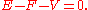The term "toroidal polydron" is also used for higher genus polyhedra and for immersions of toroidal polyhedra.

## Automorphisms

The homeomorphism group
Homeomorphism group
In mathematics, particularly topology, the homeomorphism group of a topological space is the group consisting of all homeomorphisms from the space to itself with function composition as the group operation. Homeomorphism groups are very important in the theory of topological spaces and in general...

(or the subgroup of diffeomorphisms) of the torus is studied in geometric topology
Geometric topology
In mathematics, geometric topology is the study of manifolds and maps between them, particularly embeddings of one manifold into another.- Topics :...

. Its mapping class group
Mapping class group
In mathematics, in the sub-field of geometric topology, the mapping class groupis an important algebraic invariant of a topological space. Briefly, the mapping class group is a discrete group of 'symmetries' of the space.-Motivation:...

(the group of connected components) is isomorphic to the group GL(n, Z) of invertible integer matrices, and can be realized as linear maps on the universal covering space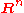that preserve the standard lattice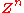(this corresponds to integer coefficients) and thus descend to the quotient.

At the level of homotopy and homology, the mapping class group can be identified as the action on the first homology (or equivalently, first cohomology, or on the fundamental group
Fundamental group
In mathematics, more specifically algebraic topology, the fundamental group is a group associated to any given pointed topological space that provides a way of determining when two paths, starting and ending at a fixed base point, can be continuously deformed into each other...

, as these are all naturally isomorphic; note also that the first cohomology group generates the cohomology algebra):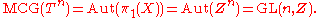Since the torus is an Eilenberg-MacLane space
Eilenberg-MacLane space
In mathematics, an Eilenberg–MacLane spaceSaunders Mac Lane originally spelt his name "MacLane" , and co-published the papers establishing the notion of Eilenberg–MacLane spaces under this name. In mathematics, an Eilenberg–MacLane spaceSaunders Mac Lane originally spelt his name "MacLane" (without...

K(G, 1), its homotopy equivalences, up to homotopy, can be identified with automorphisms of the fundamental group); that this agrees with the mapping class group reflects that all homotopy equivalences can be realized by homeomorphisms – every homotopy equivalence is homotopic to a homeomorphism – and that homotopic homeomorphisms are in fact isotopic (connected through homeomorphisms, not just through homotopy equivalences). More tersely, the map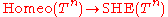is 1-connected
N-connected
In the mathematical branch of algebraic topology, specifically homotopy theory, n-connectedness is a way to say that a space vanishes or that a map is an isomorphism "up to dimension n, in homotopy".-n-connected space:...

(isomorphic on path-components, onto fundamental group). This is a "homeomorphism reduces to homotopy reduces to algebra" result.

Thus the short exact sequence of the mapping class group splits (an identification of the torus as the quotient ofgives a splitting, via the linear maps, as above):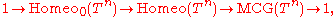so the homeomorphism group of the torus is a semidirect product
Semidirect product
In mathematics, specifically in the area of abstract algebra known as group theory, a semidirect product is a particular way in which a group can be put together from two subgroups, one of which is a normal subgroup. A semidirect product is a generalization of a direct product...

,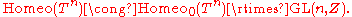The mapping class group of higher genus surfaces is much more complicated, and an area of active research.

## Coloring a torus

If a torus is divided into regions, then it is always possible to color the regions with no more than seven colors so that neighboring regions have different colors. (Contrast with the four color theorem
Four color theorem
In mathematics, the four color theorem, or the four color map theorem states that, given any separation of a plane into contiguous regions, producing a figure called a map, no more than four colors are required to color the regions of the map so that no two adjacent regions have the same color...

for the plane
Plane (mathematics)
In mathematics, a plane is a flat, two-dimensional surface. A plane is the two dimensional analogue of a point , a line and a space...

.)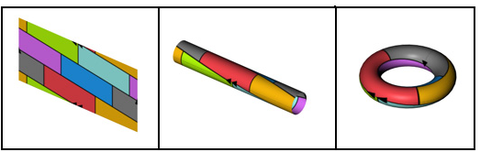## Cutting a torus

A standard torus (specifically, a ring torus) can be cut with n planes into at most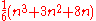parts.

The initial terms of this sequence for n starting from 1 are:
2, 6, 13, 24, 40, … .

## See also

• Algebraic torus
Algebraic torus
In mathematics, an algebraic torus is a type of commutative affine algebraic group. These groups were named by analogy with the theory of tori in Lie group theory...

• Annulus (mathematics)
Annulus (mathematics)
In mathematics, an annulus is a ring-shaped geometric figure, or more generally, a term used to name a ring-shaped object. Or, it is the area between two concentric circles...

• Clifford torus
Clifford torus
In geometric topology, the Clifford torus is a special kind of torus sitting inside R4. Alternatively, it can be seen as a torus sitting inside C2 since C2 is topologically the same space as R4...

• Complex torus
Complex torus
In mathematics, a complex torus is a particular kind of complex manifold M whose underlying smooth manifold is a torus in the usual sense...

• Dupin cyclide
• Elliptic curve
Elliptic curve
In mathematics, an elliptic curve is a smooth, projective algebraic curve of genus one, on which there is a specified point O. An elliptic curve is in fact an abelian variety — that is, it has a multiplication defined algebraically with respect to which it is a group — and O serves as the identity...

• Irrational cable on a torus
• Joint European Torus
Joint European Torus
JET, the Joint European Torus, is the largest magnetic confinement plasma physics experiment worldwide currently in operation. Its main purpose is to open the way to future nuclear fusion experimental tokamak reactors such as ITER and :DEMO....

• Loewner's torus inequality
Loewner's torus inequality
In differential geometry, Loewner's torus inequality is an inequality due to Charles Loewner. It relates the systole and the area of an arbitrary Riemannian metric on the 2-torus.-Statement:...

• Maximal torus
Maximal torus
In the mathematical theory of compact Lie groups a special role is played by torus subgroups, in particular by the maximal torus subgroups.A torus in a Lie group G is a compact, connected, abelian Lie subgroup of G . A maximal torus is one which is maximal among such subgroups...

• Period lattice
• Sphere
Sphere
A sphere is a perfectly round geometrical object in three-dimensional space, such as the shape of a round ball. Like a circle in two dimensions, a perfect sphere is completely symmetrical around its center, with all points on the surface lying the same distance r from the center point...

• Spiric section
Spiric section
In geometry, a spiric section, sometimes called a spiric of Perseus, is a quartic plane curve defined by equations of the form^2=dx^2+ey^2+f....

• Standard torus
• Surface
Surface
In mathematics, specifically in topology, a surface is a two-dimensional topological manifold. The most familiar examples are those that arise as the boundaries of solid objects in ordinary three-dimensional Euclidean space R3 — for example, the surface of a ball...

• Toric section
Toric section
A toric section is an intersection of a plane with a torus, just as a conic section is the intersection of a plane with a cone.-Mathematical formulae:In general, toric sections are fourth-order plane curves of the form...

• Toric variety
• Toroid (geometry)
Toroid (geometry)
In mathematics, a toroid is a doughnut-shaped object, such as an O-ring. Its annular shape is generated by revolving a plane geometrical figure about an axis external to that figure which is parallel to the plane of the figure and does not intersect the figure...

• Torus knot
Torus knot
In knot theory, a torus knot is a special kind of knot that lies on the surface of an unknotted torus in R3. Similarly, a torus link is a link which lies on the surface of a torus in the same way. Each torus knot is specified by a pair of coprime integers p and q. A torus link arises if p and q...

• Umbilic torus
Umbilic Torus
The umbilic torus is a single-edged 3-dimensional figure created by Helaman Ferguson as a mathematical artwork. Ferguson created a 27-inch bronze sculpture, Umbilic Torus, and it is his most widely known piece of art. The lone edge goes three times around the ring before returning to the starting...

• Villarceau circles
Villarceau circles
In geometry, Villarceau circles are a pair of circles produced by cutting a torus diagonally through the center at the correct angle. Given an arbitrary point on a torus, four circles can be drawn through it. One is in the plane parallel to the equatorial plane of the torus. Another is...

## External links

• Creation of a torus at cut-the-knot
Cut-the-knot
Cut-the-knot is a free, advertisement-funded educational website maintained by Alexander Bogomolny and devoted to popular exposition of many topics in mathematics. The site has won more than 20 awards from scientific and educational publications, including a Scientific American Web Award in 2003,...

• "4D torus" Fly-through cross-sections of a four dimensional torus.
• "Relational Perspective Map" Visualizing high dimensional data with flat torus.
• "Torus Games" Free downloadable games for Windows and Mac OS X that highlight the topology of a torus.
• Polydos
The source of this article is wikipedia, the free encyclopedia.  The text of this article is licensed under the GFDL.